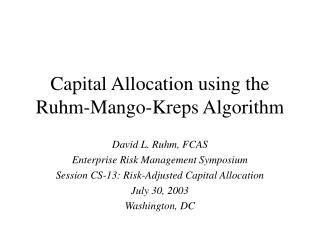DownloadDownload PresentationCapital Allocation using the Ruhm-Mango-Kreps Algorithm

# Capital Allocation using the Ruhm-Mango-Kreps Algorithm

Télécharger la présentation## Capital Allocation using the Ruhm-Mango-Kreps Algorithm

- - - - - - - - - - - - - - - - - - - - - - - - - - - E N D - - - - - - - - - - - - - - - - - - - - - - - - - - -
##### Presentation Transcript

1. Capital Allocation using the Ruhm-Mango-Kreps Algorithm David L. Ruhm, FCAS Enterprise Risk Management Symposium Session CS-13: Risk-Adjusted Capital Allocation July 30, 2003 Washington, DC

2. The Capital Allocation Problem • How can total-company capital, and its costs, be allocated to all sources of risk in a way that: • Is internally consistent (summing within parts), • Allocates in proportion to risk contributed, • Attributes diversification benefits to sources, • Can be used with any specified risk measure, • Is consistent with established financial theory.

3. The RMK algorithm RMK meets the above requirements, plus: • Is simple to use, • Can be explained, • Has solid underlying mathematical theory, • Evaluates all risk in terms of total-company, “top-down” view (instead of evaluating each part as if stand-alone)

4. The RMK Algorithm Central principle Each component is evaluated, to measure its contribution to total-company risk.

5. The RMK Algorithm • The algorithm, in short: • Simulate possible outcomes, by component and total company. Calculate unweighted average outcome (expected value) for each. • Select a risk measure on total company outcomes, expressed as higher weights on adverse outcomes. • Apply the risk-weights to the individual components, and calculate risk-weighted averages. • Allocate capital in proportion to risk, as measured by the difference between the risk-weighted average and the unweighted average: Risk ~ Risk-Weighted Expected Value – Unweighted Expected Value

6. Selecting a risk measure • Any standard risk measure (e.g., value at risk, tail-value at risk, default rate of surplus) can be expressed in the form of weights. • Simplest: Net loss outcomes > 1 , net gain outcomes = 1. • Measures tail of distribution, all loss outcomes equally weighted. • Risk Measure ~ Frequency of Loss x Average Severity of Loss. • Is a good risk measure, similar to TVaR. • Weights could be refined to distinguish among loss, gain levels. • In general, risk measure weights are: • Non-negative, • Higher for worse (“riskier”) outcomes, • Lower for better outcomes.

7. Some examples

8. RMK useful properties • Any additive allocation method can be replicated using the RMK framework. • Works with user’s choice of risk measure. • Allocates total company risk to parts, down to any desired level of detail. • Consistent with financial theory, and arbitrage-free: risk-weighted averages are equivalent to “risk-neutral” valuations. • Simple, transparent.

9. Selected References • Ruhm / Mango, “A Risk Charge Calculation Based on Conditional Probability,” Bowles Symposium, Atlanta, April 2003 (submitted to NAAJ). • Kreps, “Riskiness Leverage Ratios,” Instrat working paper • Ruhm / Mango, “A Method of Implementing Myers-Read Capital Allocation in Simulation,” CAS Spring Meeting, May 2003 (submitted to CAS Forum).